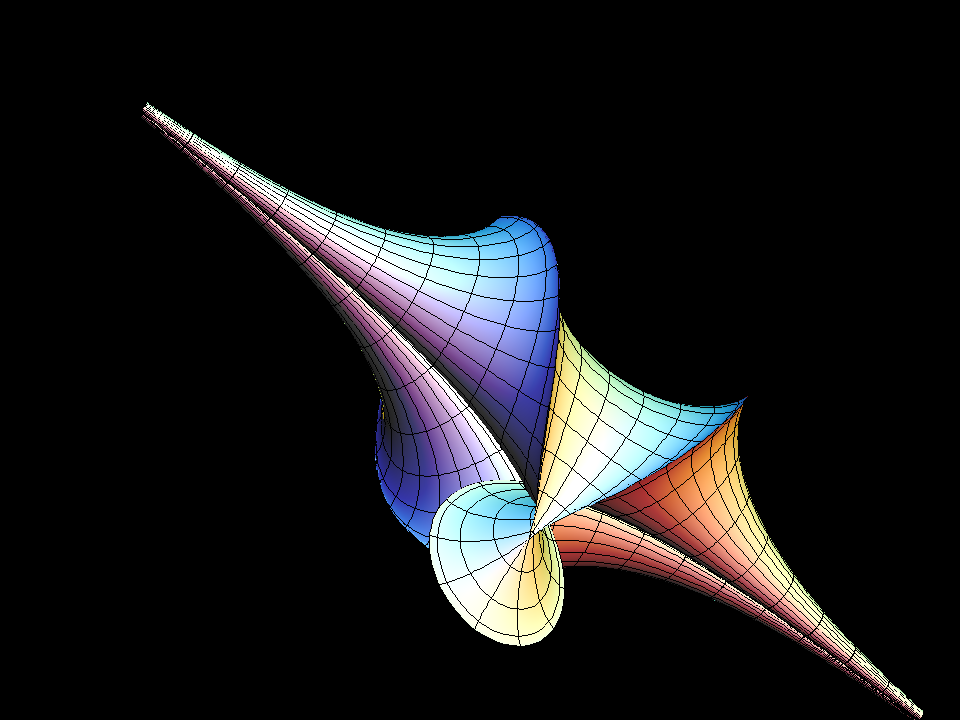# Two-Soliton SurfaceIn the 2-parameter family of 2-soliton surfaces lies this symmetric deformation of the Kuen Surface

The Two-Soliton Surface surface is the surface of Gaussian curvature K = -1 that corresponds to the following solution of the Sine-Gordon equation:

`q(u,v) := 4 * arctan( B * (exp(A2(u, v)) - exp(A1(u, v))) / (1 + exp(A1(u, v) + A2(u, v))));`

where:

```B := (cos(bb * π) - cos(aa * π)) / (cos((bb - aa) * π) - 1);

A1 := cosec(aa * π) * u - cotan(aa * π) * v;

A2 := cosec(bb * π) * u - cotan(bb * π) * v;```

Let us summarize, how the more complicated pseudospherical surfaces are constructed. If one parametrizes a surface of Gauss curvature K = -1 in special (so called “asymptotic”) coordinates, then the angle `q(x,t)` between the parameter lines determines the so called first and second fundamental forms of the surface. Once these forms are given, the surface can be obtained by solving (numerically if necessary) a pair of ODEs for the parameter lines. The fundamental theorem of surface theory says: a necessary and sufficient condition for this construction is, that the two fundamental forms satisfy the Gauss- and Codazzi- integrability conditions. In the case of our special asymptotic coordinates these integrability conditions simplify enormously to:

“The angle function `q(x,t)` must satisfy the Sine-Gordon equation (SGE)”

, which is: `d/dt d/dx q(x,t) = sin(q(x,t))`

A second function `Q(x,t)` is called a “Bäklund transformation with parameter s” if `Q(x,t)` satisfies a pair of first order equations:

• `dQ/dx(x,t) = +dq/dx(x,t) + 2 s * sin( (Q+q)(x,t)/2 )`
• `dQ/dt(x,t) = -dq/dt(x,t) + 2/s * sin( (Q-q)(x,t)/2 )`

`Q(x,t)` can be found from these two ODEs, if `q(x,t)` is an SGE solution and then `Q(x,t)` is also an SGE solution. If one starts with `q(x,t) = 0` then one obtains `Q(x,t) = 4 * arctan(exp(s*x + t/s))` . From `Q(x,t)` the Dini Surface are obtained.

An SGE marvel is that any pair s1, s2 of Bäklund parameters (with SGE solutions `Q1(x,t)` , `Q2(x,t)` allow to explicitly give another SGE solution `Qq(x,t)` , namely: `tan( (Qq(x,t) - q(x,t))/4 ) = (s1+s2)/(s1-s2)*tan ( (Q1(x,t) - Q2(x,t))/4 )` . The 2-Soliton solutions are obtained by choosing any real pairs `s = s1` , `s = s2` (with s the parameter in the Bäcklund transformation pairs of ODEs above). The construction also works with complex parameters `s1 = z, s2 = conj(z)` .

In this case Q1 and Q2 are not real functions, but Qq turns out to be real again. If `|z| = 1` , one gets the surfaces called “breathers” and for other z one gets deformations of breathers like the image above.